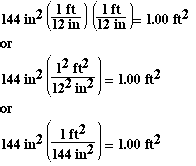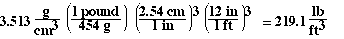### Determine the number of ft2 in 144 in2.### This problem hasw proved to be a little challenging for many students, so lets take it apart and look at it a little more carefully. We are asked to convert 144 in2 to ft2. We know a conversion between inches and feet, 12 inches = 1 foot, but how do we handle in2? As you can see in the solution we set up the factor so inches cancel, but we must square the factor. This is very important. The square means that all units and all numbers within the bracket must be squared. So the answer could also be written as,### So here is the approach we would use;